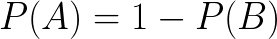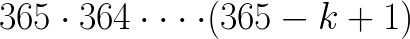I remember it like it was yesterday, I had just arrived in Dublin to kick off my study abroad program and we were filing into the bus. Attendance kicked off to make sure we hadn’t missed any stragglers — all 30 of us were present. Next, the token zany instructor — which mind you, all study abroad programs have — insisted we run through a little experiment.

“As an Icebreaker, I want you to find others born in the same month, and see if you can find someone born on the same day”

Now, I absolutely hate icebreakers, but this one intrigued me because it seemed very unlikely that there would be two people born on the same day — after all, there are 365 different possibilities, and only 30 of us. Cut to five minutes of intermingling later, and it turns out there were, in fact, two people born on the exact same day. I stared in disbelief at the zany instructor — was he a wizard? Nope, as we shall soon see — it was just a matter of probability.

It’s probably not best-practice to graph the answer to begin with, but here we are.. The above graph maps out the probability of at least two people sharing the same birthday given the number of people in the room (represented by k)

Intuitively, as the number increases, the probability of a ‘birthday match’ also increases. This makes sense — if there were only two people in the room, it would be very unlikely, but if there were 366 people in the room, then it is literally guaranteed (k > number of days in a year).

Mathematically, a 50% probability occurs at 23 people. This means my zany instructor could know with relatively high confidence that there was a chance greater than 50% that there would be a match within our bus of 30 people. Why is this? — let’s start at the beginning.

Mapping out the probability

I find it easiest to explain problems by scaling the numbers so that it can be expressed in an intuitive way.

Instead of days in the year (365), let’s scale down the numbers to a hypothetical situation:

After careful vetting, you have selected three people who you know were born on either a Monday, Tuesday, or Wednesday. You researched and obtained their birth certificate to confirm this is the case.

Now, how many different combinations of birthdays can occur? Let’s start by using brute force (write out all the possibilities).

So…as you can see, there are 9 possibilities that can occur. Not-coincidently, this is the same answer that would occur if you did calculated 3³. This gives you the number of possible outcomes

number of possible days (n) to the power of the number of people in the room (k)

This means that if there are 365 possible days, and 30 people in the room, then the number of possible scenarios is:

which is roughly 7.3924081e+76 (a giant number) so there is an insane amount of possible scenarios. which makes sense…every single one of the individuals in the room can have a birthday residing on any of the 365 days. For each person residing on a particular day (e.g. January 1st) there are 29 other people (with 365 options) which can alter the results — creating a new scenario..hopefully that makes sense.

So, of a group of 30 people, we know that there are 365³⁰ different ways their birthdays can be arranged.

Now measuring the probability of at least two people having the same birthday is difficult, because ‘at least two’, could be two, three, four, five, etc. people all having the same birthday. It is much much easier to examine the inverse probability: the probability of nobody sharing a birthday.

Now the probability of at least two birthdays is comparable to the probability of 1- the probability of nobody sharing a birthday (inverse probability). If there are two possible events, then the likelihood of one occurring is the same as the likelihood of the other not occurring.where A is the first event, and B is the inverse event

E.g. the probability of rolling a die and getting three vs. the probability of rolling a die and not getting three

Probability of rolling 3 is 1/6; the probability of not rolling 3 is 5/6

1- 5/6 = 1/6 = probability of rolling a three.

Calculating the Inverse Probability

If there are no people sharing a birthday, this means that if Person 1 is born January 1st, then no other person is born January 1st. As a result, as each person reveals their birthday, that birthday is removed from the realm of possibilities for the remaining people: the pot of possibilities for all remaining people shrinks.

Person 1 has 365 possible scenarios, Person 2 has 364, Person 3 has 363, and so on and so forth.

So rather than 365³⁰ which can also be written as:

365 x 365 x 365 x 365 x 365 x 365 x 365 x 365 x 365 x 365 x 365 x 365 x 365 x 365 x 365 x 365 x 365 x 365 x 365 x 365 x 365 x 365 x 365 x 365 x 365 x 365 x 365 x 365 x 365 x 365

(365 multiplied by itself 30 times)

the possible scenarios for people not having the same birthday is:

365 x 364 x 363 x 362 x 361 x 360 x 359 x 358 x 357 x 356 x 355 x 354 x 353 x 352 x 351 x 350 x 349 x 348 x 347 x 346 x 345 x 344 x 343 x 342 x 341 x 340 x 339 x 338 x 337 x 336

which = 2.1710302e+76 (again another big number, but not as big — which makes sense because we have limited the potential options by stating a rule that nobody can be born on the same day)

The easiest way of expressing this is:365 x 364 x …. until you get to (365-k +1)

Okay so we now have our building blocks — we know how many scenarios occur when nobody is born on the same day, and we know how many scenarios occur when there are no rules (i.e. anybody can be born on any day)

Scenarios where nobody is born on the same day:

Scenarios where anybody can be born on any day (all possible scenarios):

So similar to rolling the die where the probability = the number of scenarios where an event can occur (e.g. you roll a three— this happens in one scenario) divided by the number of all possible scenarios (six possibilities when rolling a die).. the same formula applies for the birthday problem

using a calculator the answer is

This means there is a 29% chance that nobody in the room of 30 shares the same birthday.

So there is a 71% chance that in a room of 30, there will be at least two people sharing the same birthday. The instructor wasn’t a wizard, he just knew his math.

Examing this image again, we see that this corresponds to the graph. If you were to crunch the numbers for 23 students, you would find that the probability is 50%

^^Here is a birthday simulator if you’re interested in testing it out. Note that the more trials you do, the more precise the experimental probability becomes..this becomes probability is the likelihood, not a guarantee.. there are still anomalies that can occur.

Written by

Benchmarked Path

On a journey to master data science…starting from scratch. I’ll be posting along the way to help my absorption, and hopefully, help you too.

Welcome to a place where words matter. On Medium, smart voices and original ideas take center stage - with no ads in sight. Watch
Follow all the topics you care about, and we’ll deliver the best stories for you to your homepage and inbox. Explore
Get unlimited access to the best stories on Medium — and support writers while you’re at it. Just \$5/month. Upgrade